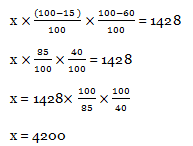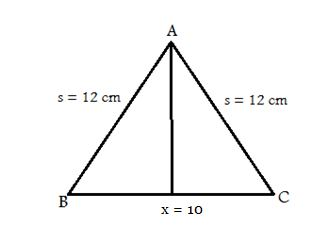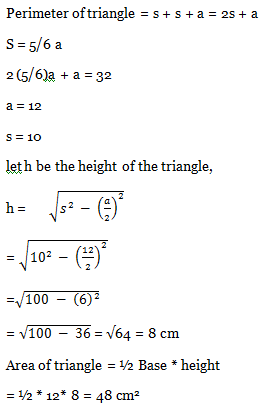# SSC CGL EXAMS 2018 | Quantitative Aptitude Practice Questions (Day-16)

Dear Aspirants, Here we have given the Important SSC Exam 2018 Practice Test Papers. Candidates those who are preparing for SSC 2018 can practice these questions to get more confidence to Crack SSC 2018 Examination.

[WpProQuiz 4664]

1) How many numbers are there from 300 to 650 which are completely divisible by both 5 and 7?

a) 8

b) 9

c) 10

d) 12

2) Nive can complete a work in 2/5 days in 6 days and Vino can complete the 1/6th of the same work in 5 days. Then they work together how many days work will get complete?

a) 5 days

b) 7 ½ das

c) 10 days

d) 15 days

3) Rs. 11700 is divided among Pravin, Preti, and Priya in the ratio of ½ : 1/3 : ¼ respectively. What is the difference between Pravin and priya?

a) 2500

b) 2600

c) 2000

d) 2700

4) If a certain number is reducing 60% and 15 % respectively, then the number is 1428, Find the number.

a) 4500

b) 3789

c) 4200

d) 6579

5) If x + 1/x  = 2, then what is the value of x81 + x 144 ?

a) – 1

b) 0

c) – 2

d) 2

6) The perimeter of an isosceles triangle is 32 cm and each of the equal sides is 5/6 times of the base. What is the area (in cm2) of the triangle?

a) 48 cm2

b) 50 cm2

c) 52 cm2

d) 54 cm2

7) After two successive discount of 10% and 15%, an article is sold after for 34425. What is the marked price of the article?

a) 55000

b) 50400

c) 45000

d) 65098

8) Five years ago the average age of 4 members in a family was 25 years. At present, a family blessed with a girl baby, but the average age of the family remains same. What age of the child?

a) 2

b) 3

c) 4

d) 5

9) If the diameter of a Sphere is 28 cm, then what is the surface area of the sphere?

a) 2464 cm2

b) 2004 cm2

c) 2460 cm2

d) 2064 cm2

10) How many times the keys of a type writer have to be pressed in order to write from 121 to 1346?

a) 1441

b) 4025

c) 1564

d) 1907

The least common multiple of 7 and 5 is 35. Therefore the numbers that you want are the multiples of 35 between 300 and 650.

300 / 35 = 9

650 / 53 = 18

35th multiple after 300 = 315 = 31*9

35th multiple before 650 = 630 = 31*18

So the multiples of 35 in the range of 300 to 650 are 35*9, 35*10, and so on until 35*18. So, 18–9 + 1 (the +1 is to include the last number) gives us 10 numbers.

So total numbers which are divisible by 35 in between 300 & 650 are = 18 – 9+1 = 10.

2/5 work = 1/6 days

1 work = 1/6 * 5/2 = 15 days

Nive’s Full work completed by 15 days

Vino’s 1/6 work completed in 5 days

1/6 work = 1/5 days

1 work = 30 days

Nive + Vino = 1/15 + 1/ 30 = 3/30 = 1/10 days

So they can work together complete the work completed in 10 days.

Total amount = Rs. 11700

Ratio of Pravin, Preti and Priya

½ : 1/3 : ¼

LCM of 2,3,4 = 12

Ratio of Pravin, Preti and Priya = 6: 4: 3

6x + 4x + 3x = 11700

13x = 11700

x = 900

Difference of Pravin and Priya = 6x – 3x = 3x

= 3* 900 = 2700x + 1/x = 2

A s we can substitute x = 1

x + 1/x = 1 + 1 = 2

x 81 + x 121 = 181 + 1144 = 1 + 1 = 2Marked price = Rs. X

x * (100/90) * (100/85) = 34425

x = 34425*(100/90) (100/85)

x = 45000

5 years ago average of 4 members = 25

Total age of the family 5 years ago = 25 * 5 = 100

At present total age of the family = 120

At present Total Age of the family including the child = 25 * 5 = 125

Age of the child = 125 – 120 = 5 years

Diameter = 28 cm

Radius = d/2 = 28/2 = 14 cm

Surface area of the sphere = 4πr2

= 4 * (22/7) * 14 * 14 = 2464 cm2

Number of times the keys have to be pressed to type numbers from 121 to 1346
= 3 (999 – 121 + 1) + 4 (1346 – 1000 + 1)
= 3* 879 + 4* (347)
= 2637 + 1388 = 4025

### *******************

#### SSC CGL EXAMS 2018 |Quantitative Aptitude Practice Questions (Day-13)